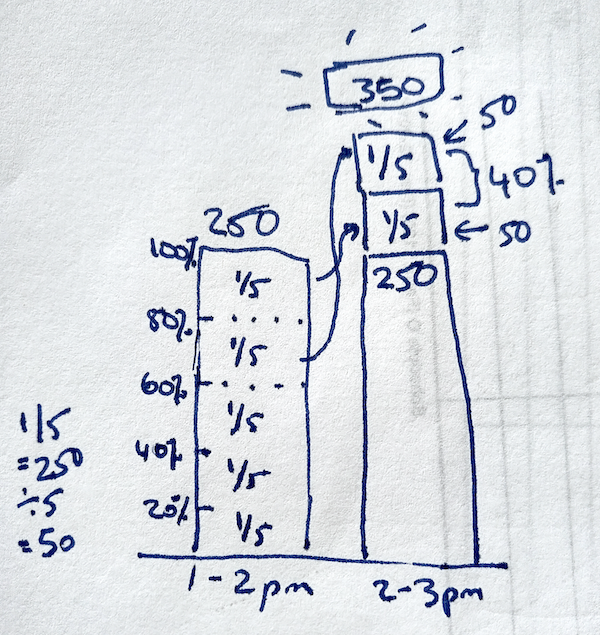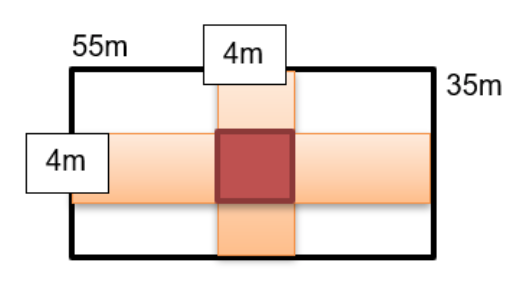# Numerical Reasoning Test – “How to Pass” Guide

Get top scores with 5 example questions & solutions + easy-to-follow numerical reasoning tips & free practice questions, developed by experts.

## What’s Numerical Reasoning?

High-paying employers and elite schools (with selective entry or scholarships) are increasingly turning to numerical reasoning tests as a part of aptitude testing to select top candidates.

Employers and school principals are not interested in inviting people who can display the results of rote learning. They’re looking for employees, students or graduates who can understand worded problems, and apply mathematical concepts to real-life situations and solve problems.

The competition is fierce so it’s necessary to prepare for your numerical reasoning test in order to pass your first hurdle.

So… what exactly is the definition of a numerical reasoning test and importantly, how do you pass it?

### Numerical Reasoning Test - Definition, Format, Time Limit & Challenges

#### A numerical reasoning exam is one that tests how quickly and accurately you reason and solve problems with numbers.

Numerical reasoning test questions can be broadly categorised into three main categories:

• number sequences and series – this type tests your logic and ability to see the relationship between numbers, aka “patterns” to solve the problem.
• worded problems – this question type requires you to work through problems expressed in prose.
• interpretation of data from graphs and charts – this type of question tests your ability to identify trends and relationships in the data represented visually.

Numerical reasoning tests also share 3 common features:

1. Multiple-choice format - Apart from the correct option, you’ll always have 1 or 2 “distractors” – the options that the test creators throw in to distract you from the right answer. If you make a silly mistake in your calculations, these are the solutions you’ll get in the end.
2. Multi-stage calculations - You need to know which operations to do and in what order.
3. Strict time limit - think 30-40 seconds per one question.

Be ready and get your best test score with our numerical reasoning test prep - see self-paced numerical reasoning course containing 100+ practice questions and teaching test banks to learn all the shortcuts!

## 5 Easy-to-Remember Numerical Reasoning Test Formulas & Tips To Make The Most Out Of Your Preparation

It doesn’t matter whether you’re taking an SHL, Cubik’s, or any other type of numerical reasoning test. They will all challenge your time management skills and put you under extreme pressure.

Whether you’re taking the numerical reasoning test online or on paper, whether you’re a job candidate or school candidate, there’s only one thing that matters.

Effective test preparation.

By effective, I don’t mean how many hours you spent preparing but rather, whether you can use what you’ve learnt to answer new questions.

Here are 5 simple and actionable numerical reasoning test formulas and tips (using similar-to-the-exam sample questions) to make your preparation as effective as possible.

### Numerical Test Tip 1 - Round up/down to do calculations quickly

The question below requires you to do calculation, but calculating numbers like 5%, 14% or 27% directly may take some time. Shorten your calculation time by rounding up/down and then working your way to the correct number. For example, in the question below, calculate 10% as that can be done easily and then divide by 2 (to get 5%).

Try the question yourself and then see how we answer the question quickly, in 5 steps or less.

#### Question 1

If a recipe book costs \$120 and a pen costs 95% less than the recipe book, how much does the pen cost?

A: \$5 B: \$6 C: \$7 D: \$4 E: \$10

#### Solution:

Step 1: Find the cost of the pen

To find the cost of the pen we need to calculate 5% of \$120. This is because the book costs \$120 and if the pen is 95% less, then it is also 5% of the book (100% - 95%, note that 100 is 100% being the full cost (i.e. the full cost of the book) as we’re working in percentages here).

Step 2: Solve through rounding up/down

We can solve the problem in a matter of seconds, mentally, by doing 10% of \$120 (\$12) and then dividing by 2 (\$12 / 2 = 6) to get the answer 6, which is 5% of 120. When you’re multiplying by ten or dividing by 10 all you have to do is move the decimal point. The correct answer is B.

Ready to start on practice questions yourself? Learn how to answer questions quickly with full explanations and step-by-step solutions through our online numerical teaching test bank.

### Numerical Test Tip 2 - Depict the problem visually to help make problem solving easier

What many find hard in numerical reasoning isn’t the calculations but figuring out what to calculate in the first place. Depicting the problem visually helps make this easier. See how we do this in Question 2 below.

#### Question 2

The shop received its highest number of orders between 2 p.m. and 3 p.m., which was 40% more than the 250 orders it received between 1 p.m. and 2 p.m. How many orders did the shop receive between 2 p.m. and 3 p.m.?

A: 100 B: 450 C: 350 D: 625 E: 150

#### Solution:

Step 1: Visually depict what you need to solve

We know that the shop has 250 orders and that between 2pm - 3pm it receives 40% more than the 250 orders. So let’s put this down visually to help make it easier for us to figure out what to calculate:Step 2: Calculate down, and then up, quickly

It’s quite clear from the image that 40% would be 100 so we add 100 to 250 and you get answer option C, 350.

Want to learn shortcuts like these? Learn exam shortcuts and effective strategies to beat the time limit in our numerical reasoning course.

## Numerical Test Tip 3 - Remember to take away ‘overlaps’ and/or add in for ‘overhangs’

Trickier questions in numerical reasoning questions, like those that ask you to calculate a frame or a tablecloth may actually ask you to add in calculations (one of each side of the table) in order to get to the correct answer. There are other questions where you might need to minus the overlap. Let’s see one now.

#### Question 3

A rectangular lawn 55 metres by 35 meters has two roads, each 4 metres wide running in the middle of it, one parallel to one side of the lawn while the other parallel to breadth. The cost of gravelling the roads at \$0.75 per square metres is:

A: \$422 B: \$511 C:\$420 D: \$258 E: \$32

#### Solution:

Step 1: Illustrate the concept on paperStep 2: Calculate the total square metres of the road

Since the roads intersect at the center we have to calculate that square space in the middle only once:

4x35 + 4x55 – 4x4 = 140 + 220 – 16 = 344 sq.m.

Step 3: Calculate the cost of gravelling

344 x 0.75 = \$258

Note that if we hadn’t illustrated the concept on paper, it would have been really easy to miss out on a little, yet essential detail – the fact that roads intersect.

And this means wasted time, lost scores, and lost opportunities.

### Numerical Test Tip 4 - Know which operators to use with certain words

One of the trickiest things with numerical reasoning (especially if English is not your native language) is bridging the connection between the worded question and deciding on which mathematical operators to use when doing calculations.

Here’s a cheat sheet. When a question has the word:

• Of - it generally implies multiplication.
• And - it generally implies addition.
• Parts - it generally implies division, fractions and ratios.
• Within - it generally implies fractions.
• Total - it generally implies addition.
• Difference - it generally implies subtraction.

Now, let’s see this in action through a sample practice question.

#### Question 4

A certain recipe of chocolate milk shake contains 2 parts of milk and 3 parts of chocolates per litre. In order to prepare 350 litres of the same recipe, how many litres of milk is required?

A: 150 B: 280 C: 210 D: 140 E: 235

#### Solution:

There are a lot of words being used here. Here the words “part” mean fractions of a litre, so 2 parts and (+) 3 parts is 5 parts. The word “of” here doesn’t mean you have to multiply but that the parts belong to certain groups of ingredients e.g. milk and chocolate.

Check out the video solution here:

### Numerical Test Tip 5 - Know the whole and its parts

It’s very easy to get confused with the different types of ways that parts of a whole are presented. Here are rules to remember:

• Ratios - when you have something that looks like 2:2:4, this means in total, you have 8 parts (2+2+4) of 1 whole but that whole is split unevenly between 3 people. 2 people (Person A & B) get 2 parts each and then Person C gets double of what Person A or B has.
• Fractions - this represents one part of a whole and can be expressed in multiple ways. If that part is larger than 1 whole, you’ll see something like this 1.5 of 3 / 2.
• Wholes - for percentages, the whole is expressed at 100% but it doesn’t mean that it is 100. It could be 5 cats in a basket (100%) and if 40% are sold, that means 2 cats have been sold.

Onwards now… to the practice question.

#### Question 5

Jack and John share the fish caught in the ratio of 3:5. If Jack caught 180 fish, how many did John catch?

A: 300 B: 280 C: 230 D: 180 E: None of the above

#### Solution:

Step 1: Set up the calculation formula through reasoning

3 parts and 5 parts means that in total you have 8 parts. Jack has 3 of the 8 parts, John has 5 of the 8 parts. Jack has 180 which means that each of the 8 parts is worth 60 because he has 3 of them (180 divided by 3).

Step 2: Reason to the answer

John has 5 of the 8 parts so that’s 300 (because 5 x 60), Option A. Simple right?

There are other ways you could have set up this solution. One way is this:

3/8 = 180
5⁄8 = f
f = (5⁄8 x 180 )/(3⁄8) = 900/3 = 300

But... this is longer and harder to do. The easier way is to reason and to reason well with parts, you need to understand the whole and its parts.

### Practice Online to Ace Your Job or School Aptitude Test

We’ve put in hundreds of hours designing efficient test prep tools to help you get real results. See available test preparation to help you ace your exam now.

Numerical Reasoning Test Preparation

Get all the right tools to ace your numerical reasoning test in ONE place. This self-paced online course combines comprehensive video lectures and exam strategy with ample practice to give you the maximum advantage on your important test day.

Throughout the course, you'll discover time-busting formulas, secret tips and tricks to tackle non-calculator questions and gain scholarships/selective schools entry.

Finally, you'll be able to check your knowledge with the end-of-the course exam.

Got some of the answers wrong? No worries! Questions come with in-depth explanations so you could hone your test-taking skills to perfection.
Great for:

• NSW HSPT (General Ability)
• ACER scholarships and selective schools exams
• Edutest scholarships and selective school tests like Victorian Selective Schools (MacRob, Melb High, Suzanne Cory and Nossal)
• NSW Police Force Test
• Vic Police Test and PSO Test